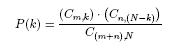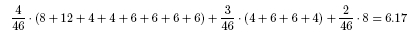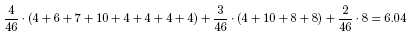# Cribbage Solutions

1. In this case, a 5,6,7,8,J, or the nine of the same suit as the jack in your hand will give you at least eight points in the show. So of the 46 cards remaining in the deck, a total of 17 will give the desired hand, giving a probability of (17/46)= 0.37.
2. There are a total of 46 cards not in your hand, so there are C46,3 = 15,180 different 3 card combinations for the starter and dealer's discard. Of the cards not in your hand, 15 have counting value 10. Therefore, the number of combinations with n cards of counting value 10 and (3-n) cards of counting value less than 10 is C15,n · C31,3-n. Therefore we have:

 Value of n 0 1 2 3 Hands with n cards of value 10 4,495 6,975 3,255 455 Probability of n cards of value 10 0.296 0.459 0.214 0.030

Therefore, the expected value of the number of counting value 10 cards is

0 · 0.296 + 1 · 0.459 + 2 · 0.214 + 3 · 0.030 = 0.978
So on average we would expect approximately one card of counting value ten.

This is an example of the more general idea of a hypergeometric probability distribution. If you have an urn containing m red balls and n green balls and you choose N of these balls at random (without replacement), then the probability that you draw exactly k of the red balls is:The denominator gives the total number of ways of choosing N balls from the n+m balls in the urn. The numerator is the product of the number of ways of choosing k of the m red balls and N-k of the n green balls, which gives the total number of ways of chooing N balls with exactly k red.

The expected value of a general hypergeometric disribution is N · m / (n+m). This is the initial probability of drawing a red ball times the number of balls that will be drawn. In our example, we are picking three cards (N=3), with 15 cards of couting value ten (n=15) and 31 cards of counting value less than 10 (m=31). So our expected value is (3 · 15)/(15+31) = 0.978.

3. First we determine the probability that our initial six card hand contains three fives and a jack, all of different suits, but not the remaining five (which needs to be the starter card). There are C52,6 = 20,358,520 different six card hands that we can be dealt. We can choose the three fives that we will be dealt in C4,3 = 4 different ways. Since the jack must be of a different suit than all of the fives, once we choose the three fives the jack is chosen as well. The final two cards in our hand can be any of the remaining cards except for the fourth five. These two cards can therefore be chosen in C47,2 = 1,081 ways. This gives a total of 4 · 1,081 = 4,324 different hands containing three fives and a jack all of different suits (but not the fourth five), giving a probability of 4,324/20,358,520 = 0.00021239 of being dealt this type of hand.

In order to score a 29, we need the starter card to be the one five not in our initial hand. There are 46 cards not in our initial hand, so the probability of the last five being the starter is 1/46 = 0.02173913. Therefore, the probability of getting a 29 is 0.00021239 · 0.02173913 = 0.000004617, or 1 in 216,580.

4. In the chart below we calculate the value of the show for each of the two hands depending on the rank of the starter card.

 Hand Ace 2 3 4 5 6 7 8 9 10 Jack Queen King 2,2,3,6 8 8 4 12 4 6 6 4 4 6 6 6 6 2,6,7,9 4 8 4 6 7 10 8 10 8 4 4 4 4

Multiplying each of these point values by the probability of the starter taking on each of the various ranks gives an expected value for the 2,2,3,6 hand ofand an expected value for the 2,6,7,9 hand ofSo while the 2,2,3,6 hand only scores two points before the starter is included and the 2,6,7,9 hand scores four points, the 2,2,3,6 hand has a greater expected value once the starter is added. In an actual game, other factors such as the value of the cards being placed into the crib, the value of cards during the play, and the current score of the game, are combined with this type of expected value combination to determine the best four cards to keep.